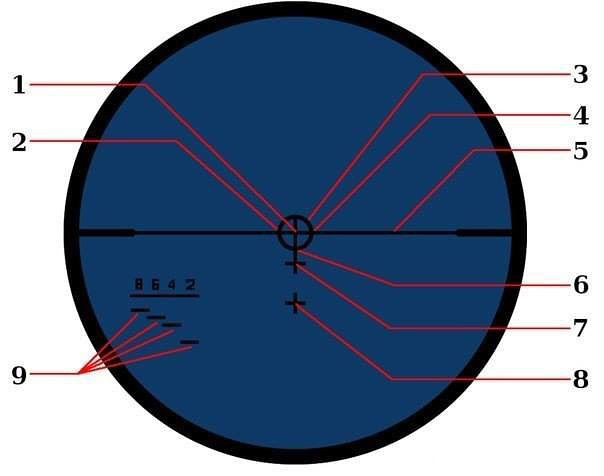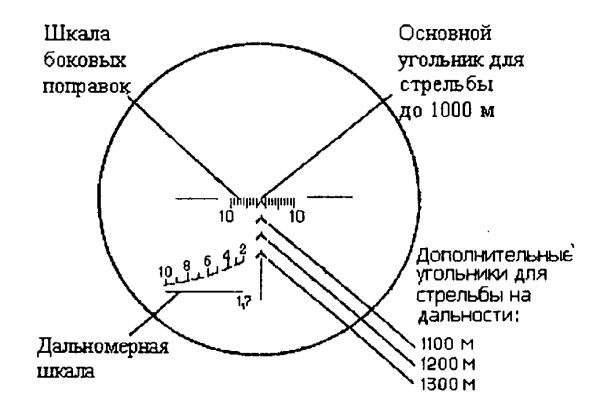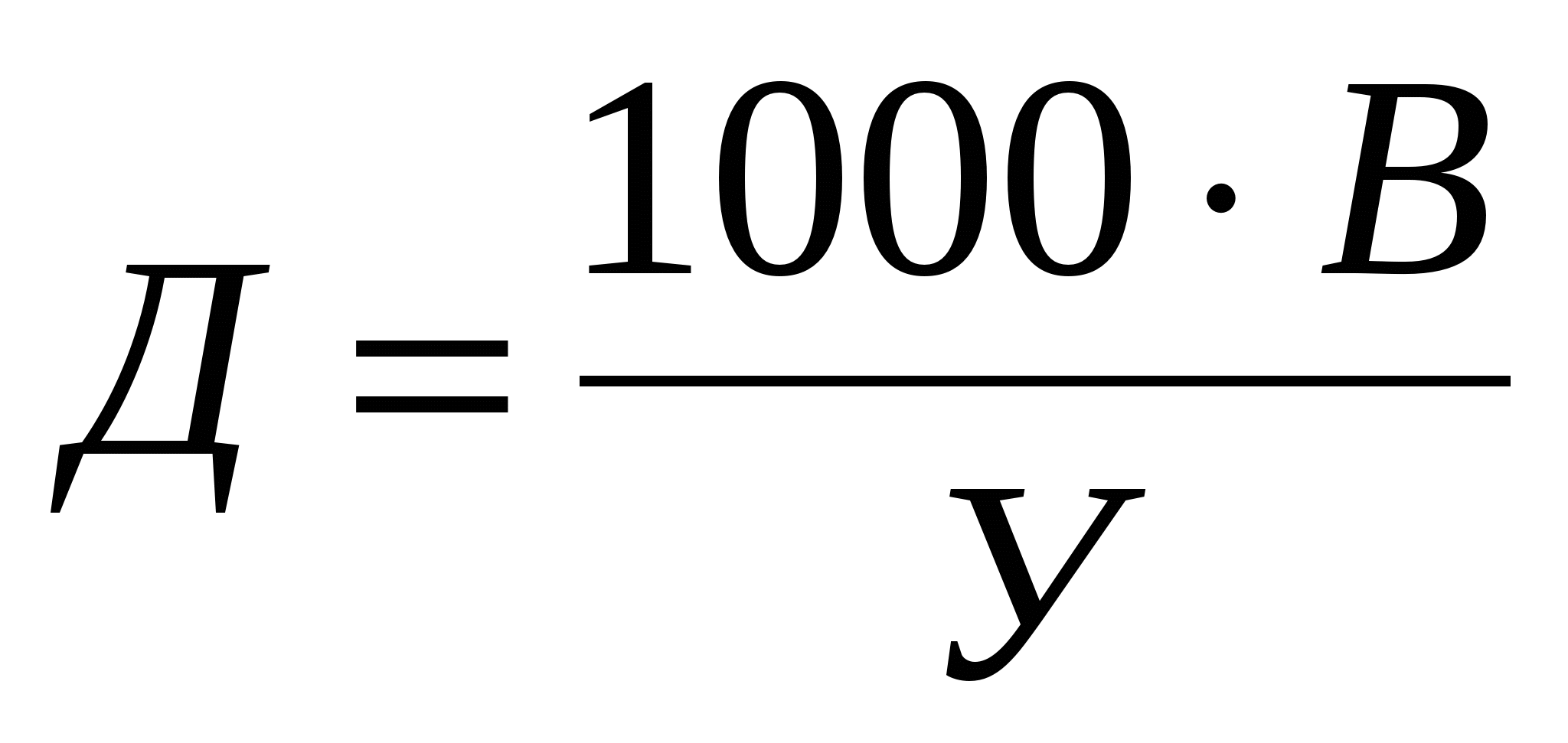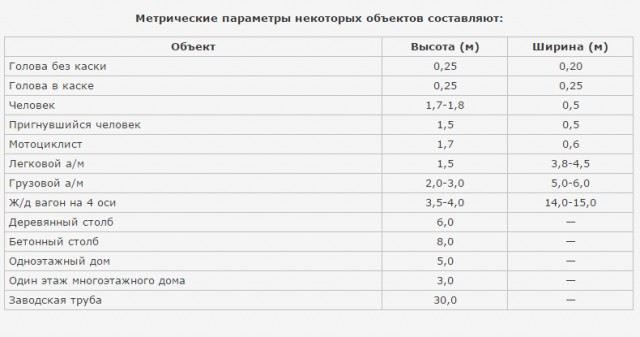This page has been robot translated, sorry for typos if any. Original content here.

# Markings on the reticle of the sniper scope1. Aiming point for shots at a distance of about 200 meters.
2. The aiming point on targets that move from left to right at a speed of about 8 km / h, a distance of about 200 meters.
3. This circle includes a person 1.75 meters tall at a distance of 400 meters.
4. The aiming point on targets that move from right to left at a speed of about 8 km / h, a distance of about 200 meters.
5. Line for determining deviation from the horizon.
6. Aiming point for shots at a distance of about 400 meters.
7. Aiming point for shots at a distance of about 600 meters.
8. Aiming point for shots at a distance of approximately 800 meters.
9. The height of a person is 1.75 meters tall at distances: 800, 600, 400 and 200 meters.Range to target = 1000 (constant value) * by the height of the object, and divide by the viewing angle.Usually human height is calculated from the calculation of 1.7 - 1.8 meters. Agree, few people with a height of 190 cm and more. Truck, armored personnel carrier - 3 meters, each floor of the building - 3 meters, etc.## Ballistic calculator

Each barrel, type of ammunition must be shot under the arrow. Otherwise, all this information becomes useless.